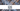# Intersection of Two Arrays-II - Leet Code Solution

September 01, 2020## Problem Statement

Given two arrays, write a function to compute their intersection.

Example

``````# Example 1:
Input: nums1 = [1,2,2,1], nums2 = [2,2]
Output: [2,2]

# Example 2:
Input: nums1 = [4,9,5], nums2 = [9,4,9,8,4]
Output: [4,9]``````

Note:

• Each element in the result should appear as many times as it shows in both arrays.
• The result can be in any order.

## Solution using Extra Memory

We need to have some sort of counter for every number that conveys what is the occurrence of that number.

For Input

``nums1 = [1,2,2,1], nums2 = [2,2]``

If we create a `HashMap<Integer, Integer>` which maintains count of each number in an array.

``````# HashMap for nums1 array
1 -> 2
2 -> 2``````

In first pass, we can populate this `HashMap`, and in second pass we can iterate over second array and compare numbers from this map. We need to decrement count of matched number on each match.

### Code

``````public int[] intersect_extraMemory(int[] nums1, int[] nums2) {
if (nums1.length == 0 || nums2.length == 0) {
return new int[] {};
}

//prepare map with number of occurence of each number
Map<Integer, Integer> map = new HashMap<Integer, Integer>();
for (int j=0; j<nums2.length; j++) {
Integer count = map.getOrDefault(nums2[j], 0);
count ++;

map.put(nums2[j], count);
}

List<Integer> res = new ArrayList<Integer>();
for (int i=0; i<nums1.length; i++) {
int count = map.getOrDefault(nums1[i], 0);
if (count > 0) {

count --;
map.put(nums1[i], count);
}
}

//copy the result array
int[] r = new int[res.size()];
for (int i=0; i<res.size(); i++) {
r[i] = res.get(i);
}

return r;
}``````

### Complexity

The code runs in `O(m + n)`, where m and n are the length of two arrays respectively.

## Solution using Sorting

If we sort the two arrays. We can start comparing the numbers from begining. Since we know the numbers are in increasing order. We can increment the indexes of the two arrays on each match and non-match.

i.e. For example, we found the number from first array is smaller than one in second array. It means, we need to increment index from first array.
Similarly if we found a match, we need to increment indexes of both the arrays.

Lets see how.

### Code

``````public int[] intersect(int[] nums1, int[] nums2) {
if (nums1.length == 0 || nums2.length == 0) {
return new int[] {};
}
Arrays.sort(nums1);
Arrays.sort(nums2);

int i=0;
int j=0;

List<Integer> res = new ArrayList<Integer>();
while (i<nums1.length && j<nums2.length) {
if (nums1[i] == nums2[j]) {
i++;
j++;
}
else if (nums1[i] < nums2[j]) {
i++;
}
else {
j++;
}
}

//copy the result array
int[] r = new int[res.size()];
for (i=0; i<res.size(); i++) {
r[i] = res.get(i);
}

return r;
}``````

### Complexity

The complexity is `O(mLog(m) + nLog(n))` due to sorting.